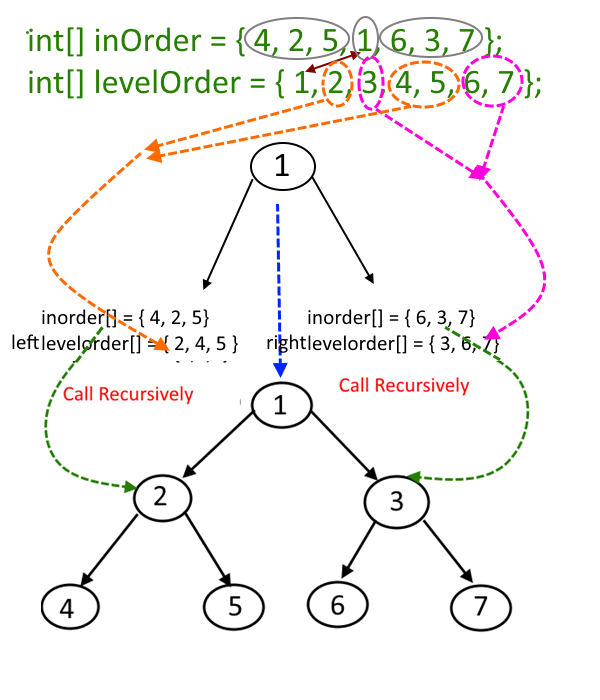# Construct a binary tree from given Inorder and Level Order Traversal

Objective: - Given an inorder and level order traversal, construct a binary tree from that.

Input: Inorder and level order traversal

Approach:

int

```[] inOrder = { 4, 2, 5, 1, 6, 3, 7 };
```

int

`[] levelOrder = { 1, 2, 3, 4, 5, 6, 7 };`
• The first element in the levelorder [] will be the root of the tree, here it is 1.
• Now the search ele­ment 1 in inorder[], say you find it at posi­tion i, once you find it, make note of ele­ments which are left to i (this will con­struct the left­sub­tree) and ele­ments which are right to i ( this will con­struct the rightSubtree).
• Suppose in the previous step, there is X number of elements which are left of 'i' (which will construct the left subtree), but these X elements will not be in the consecutive in levelorder[] so we will extract these elements from levelorder[] by maintaining their sequence and store it in an array say newLeftLevel[].
• Similarly, if there are Y number of elements which are right of 'i' (which will construct the right subtree), but these Y elements will not be in the consecutive in levelorder[] so we will extract these elements from levelorder[] by maintaining their sequence and store it in an array say newRightLevel[].
• From the previous two steps construct the left and right subtree and link it to root.left and root.right respectively by making recursive calls using newLeftLevel[] and newRightLevel[].
• See the picture for a better explanation.

[caption id="attachment_1869" align="aligncenter" width="400"]Construct-a-binary-tree-from-given-Inorder-and-Level-Order-Traversal[/caption]

Code:

Output:

```inorder traversal of constructed tree :
4  2  5  1  6  3  7
```